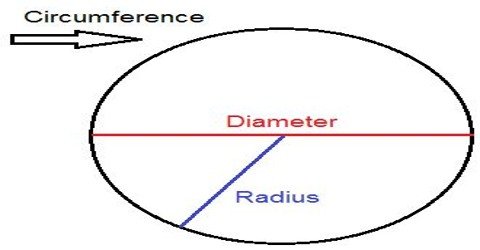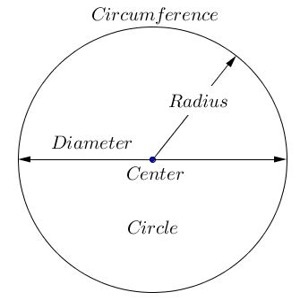Mathematic

# How to Calculate the Circumference of a Circle?Calculating the Circumference of a Circle

A circle is a shape with all points the same distance from the center. The distance around a circle is called the circumference. The distance across a circle through the center is called the diameter.

The circumference of a circle is the distance around the outside of the circle. It could be called the perimeter of the circle.A line that is drawn straight through the midpoint of a circle and that has its end points on the circle border is called the diameter (d). Half of the diameter, or the distance from the midpoint to the circle border, is called the radius of the circle (r).

How to find the circumference of a circle:

The circumference of a circle can be found by multiplying pi (π = 3.14) by the diameter of the circle.

If a circle has a diameter of 4, its circumference is (3.14 x 4) ≈ 12.56

If you know the radius, the diameter is twice as large.Circumference, diameter and radii are measured in linear units, such as inches and centimeters. A circle has many different radii and many different diameters, each passing through the center. A real-life example of a radius is the spoke of a bicycle wheel.